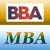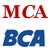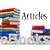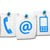Simulation in Operations Research

Simulation is a technique which describes a process by developing a model of that process, and then performing experiments on the model to predict the behaviour of the process over time.

"Models are abstractions built to understand a problem before implementing a solution ." - Anonymous

An example of simulation is in computer games (e.g., chess, field combat war games, etc.). If the sequence of events in such games were predetermined, the player would quickly learn the sequence and become bored. One solution would be to have a large number of games stored in the program, but this could take up an inordinate amount of memory space. The usual solution is for the game program to choose its own moves at random. In most games, the total number of possible combinations of events or moves is so astronomically large that this method results in each game being unique. Some other examples of a simulated environment are planetarium shows and the environments in a museum.

Experiments performed with simulation models can have many objectives like evaluating design alternatives for some new operating system, examining the effects of changes on an existing system, etc.

Definitions

Simulation starts when all else fails, i.e., it is a "Method of Last Resort".

Simulation is a technique of problem solving based upon experimentation performed on a model of real world situation.

Simulation is a numerical technique for conducting experiments on a digital computer, which involves certain types of mathematical and logical relationships necessary to describe the behaviour and structure of a complex, real world system over extended period of time.

When simulation should be used?

• Actual observation of a system may be too expensive.
• The problem is too big or intricate to handle with linear, dynamic, and standard probabilistic models.
• The standard sensitivity analysis is too clumsy and computationally burdensome for observing the actual environment.
• It is not possible to develop a mathematical model. Even though a mathematical model can be formulated, a straight forward analytical solution may not be available.
• It is not possible to perform validating experiments on mathematical models describing the system.
• There may not be sufficient time to allow the system to operate extensively.

Share and Recommend

•MBA & BBA
•BCA & MCA
•Ebooks & Articles
•Contact us